# Converting Activity Data to Impact Data

Follow

One of the important data processing steps applied by Scope 5 is the conversion of activity data to impact data. Activity data represents specific activities such as:

• using a certain amount of electricity
• consuming a certain amount of natural gas
• driving a certain distance

Impact data is derived from activity data and represents impacts or results, such as:

• the weight of specific greenhouse gas (GHG) emissions generated
• pollutants
• energy generated
• energy lost in transmission and distribution

Scope 5 quantifies all sorts of impacts associated with different activities. The most commonly considered impacts are GHG emissions.

In this article, we'll briefly discuss the general manner in which Scope 5 resources are used to convert activity data to impact data and then we'll discuss some specific examples of converting activity data to GHG emissions.

## How Resources Convert Activity Data to Impact Data

Each tracker in Scope 5 maintains an activity ledger that records the tracker's activity in the form of discrete activity records. Each activity record quantifies a specific activity over a specific time period (typically, the period between consecutive activity records). Activity from multiple activity records can be aggregated over time and prorated to specific date boundaries specified in a query. Activity can be aggregated across multiple trackers specified in a query. Activity can also be considered discretely for an individual activity record with no proration or aggregation. Regardless of whether and how activity is prorated or aggregated, any quantity of activity can be converted to impacts.

Conversion of activity data to impact data is performed separately for each tracker. Each tracker points to a Scope 5 resource that specifies how that tracker's activity data is converted to impact data. A resource may specify:

• no conversion at all
• simple conversion or complex conversions
• single or multiple conversions

### No Conversion

In many cases, users are interested in the activity data directly and no conversions are applied by the tracker's resource. Examples of activity that may not be converted include waste generated or water used. These activities can be converted to impacts but often are not.

### Simple Conversion

Almost all conversions applied by Scope 5 resources are simple conversions to one or more GHGs using one or more emissions factors. Another type of simple conversion is conversion to energy using an energy conversion factor. In general, simple conversions convert a quantity of activity to a quantity of impact by multiplying the activity by a conversion factor.

### Complex Conversions

In certain cases, resources may specify more or less complex conversion formulas or may use lookup tables to convert activity to impacts. Examples of more complex conversions include:

• A two-step conversion in which the activity data indicates a facility's area. In the first step, energy use intensity (EUI) benchmarks are first used to convert the facility's area to expected electricity or natural gas usage. In the second step, emissions factors are applied to convert the electricity or natural gas usage to emissions.
• Air-travel activity can be expressed in the form of origin/destination city pairs. In this case, a trigonometric formula may be used to calculate the great-circle distance between the two cities. That distance is then evaluated to determine whether the trip should be categorized as short, medium or long haul. Depending on the categorization, the distance flown is multiplied by one of three emissions factors to calculate the GHG emissions resulting from the trip.
• Various chemical manufacturing processes generate fugitive emissions of certain GHGs. The weight of GHGs generated might be calculated using simple conversion factors based on stoichiometry. Abatement equipment might then be used to mitigate the net emissions impact. The effect of the abatement equipment might be expressed using linear or non-linear equations.

## Common Conversions

In this section, we'll look at some specific examples of simple conversions.

### Conversion to CO2e Using a Single Emissions Factor

One of the most common conversions converts a quantity of activity data to a single quantity of GHG emissions using a single emissions factor:

Aelectricity (kWh) x EFCO2e (kg/kWh) = emissions (kg CO2e)

In this example, a unit of electric activity (expressed in kilowatt-hours) is converted to a weight of GHG (expressed in kilograms of carbon dioxide equivalent) using an emissions factor (expressed in kilogram per kilowatt-hour).

Conversion to CO2e Using Multiple Emissions Factors

Another common conversion converts a single quantity of activity to quantities of multiple GHG emissions:

CO= Aelectricity x EFCO2

CH= Aelectricity x EFCH4

N2= Aelectricity x EFN2O

CO2e = CO2 x GWPCO2 + CH4 x GWPCH4 + N2O x GWPN2O

In the first three rows above, the electricity activity is converted to three different GHG emissions impacts (CO2, CH4 and N2O) using the corresponding emissions factors. In the fourth row, those GHG impacts are then converted to their CO2 equivalent impacts using their corresponding global warming potentials (GWPs), and summed to produce the total CO2 equivalent emissions.

Given that the GWP for CO2 is '1', the equation for CO2e can be simplified to:

CO2e = CO2 + (CH4 x GWPCH4)+ (N2O x GWPN2O)

Here, we have four impacts - CO2, CH4, N2O and CO2e.

### The Numbers behind the Variables

The two sets of equations above show the algebra used to convert electricity activity to GHG emissions. Users can find specific numbers for emissions factors and global warming potentials in various locations in the Scope 5 application and in documents referenced by the application.

#### Listing of Conversion Factor Sets in Resource Library Pages

Users can inspect a tracker's resource to see the emissions factors and global warming potentials applied. To do so, either visit your account's resource library (Organization => Resource Libraries => [library of interest]) or navigate directly to the specific resource from the tracker page by clicking the link in the tracker description bar: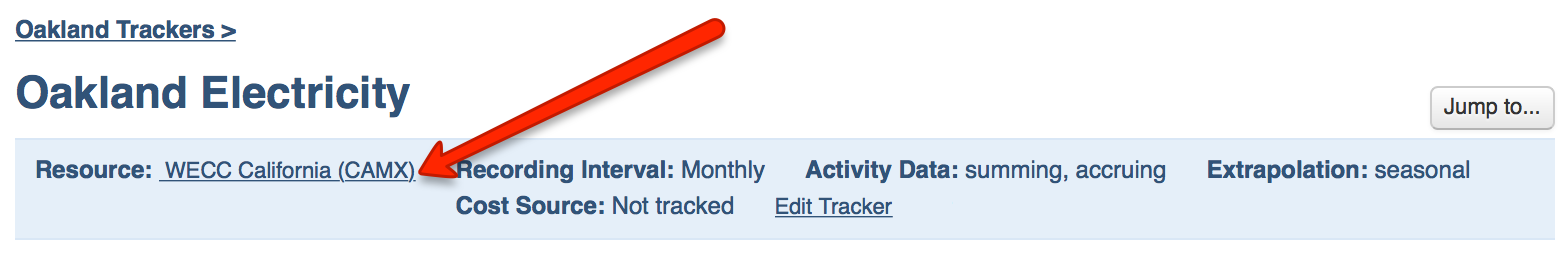This will take you directly to a table listing the resource's emissions factors: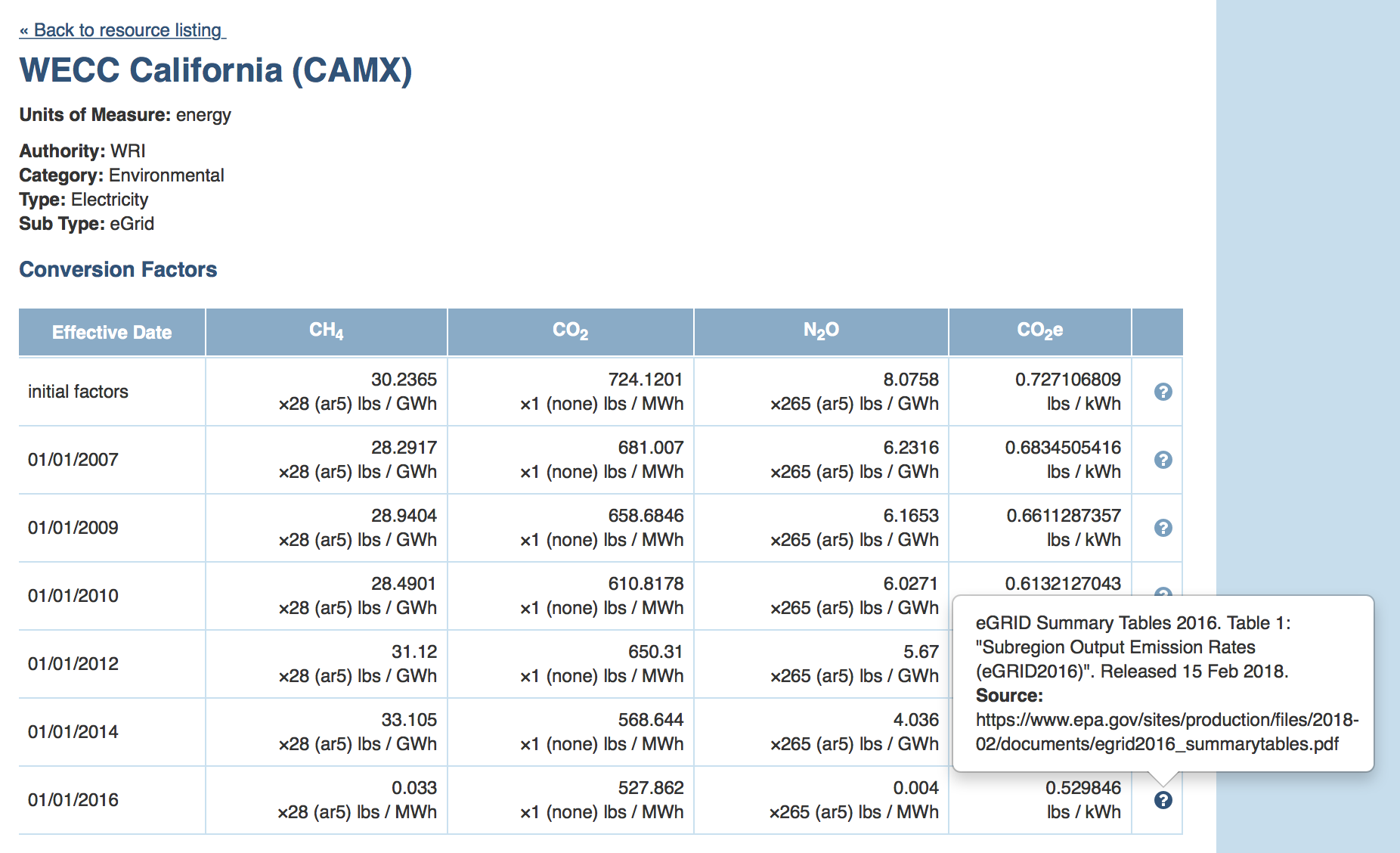There are several things to note in this screenshot.

Conversion Factor Sets

First - there are several rows of emissions factors. Each row corresponds to an emissions factor set. Many emissions factors are updated periodically by the authorities that provide the emissions factors to reflect operational changes (such as changing fuel mixes used to generate electricity). Updated emissions factors are added to the corresponding Scope 5 resource library in the form of a conversion factor set with an effective date indicating the earliest date of activity to which it should be applied. It's important to keep this in mind when considering how activity is converted to impacts. Scope 5 will automatically select the set of conversion factors or emissions factors corresponding to the date of the activity that is being converted. If the activity converted was incurred over a period that spans multiple conversion factor sets, the activity will be converted using all applicable conversion factor sets, on a prorated basis.

Note that many resources have only a single conversion factor set.

Emissions Factors and Global Warming Potentials

Each impact is quantified across all emissions factor sets in a separate column. Let's look at the 2016 emissions factor set in the last row of the table. We see that the emissions factor for CH4 is 0.033 lbs./MWh. We can also see what GWP is applied to convert the CH4 emissions to CO2e. In this case, a GWP of 28 is applied. The parenthetic text ar5 tells us that this GWP is from the IPCC's Fifth Assessment Report. In the next two columns, we see the emissions factors and GWPs for CO2 and for N2O. Note that (not surprisingly) the GWP for carbon dioxide is 1. Finally, in the fourth column, we see the conversion factor for CO2e, which is generated by the resource, using the formula above.

#### Description and Source

By hovering over any of the question mark symbols in the last column of the table, users can see a description of the methodology and the source of any numbers used in the conversion of activity to impacts. Additional information may be provided above the conversion factor table.  An example for a slightly more complex conversion is illustrated below: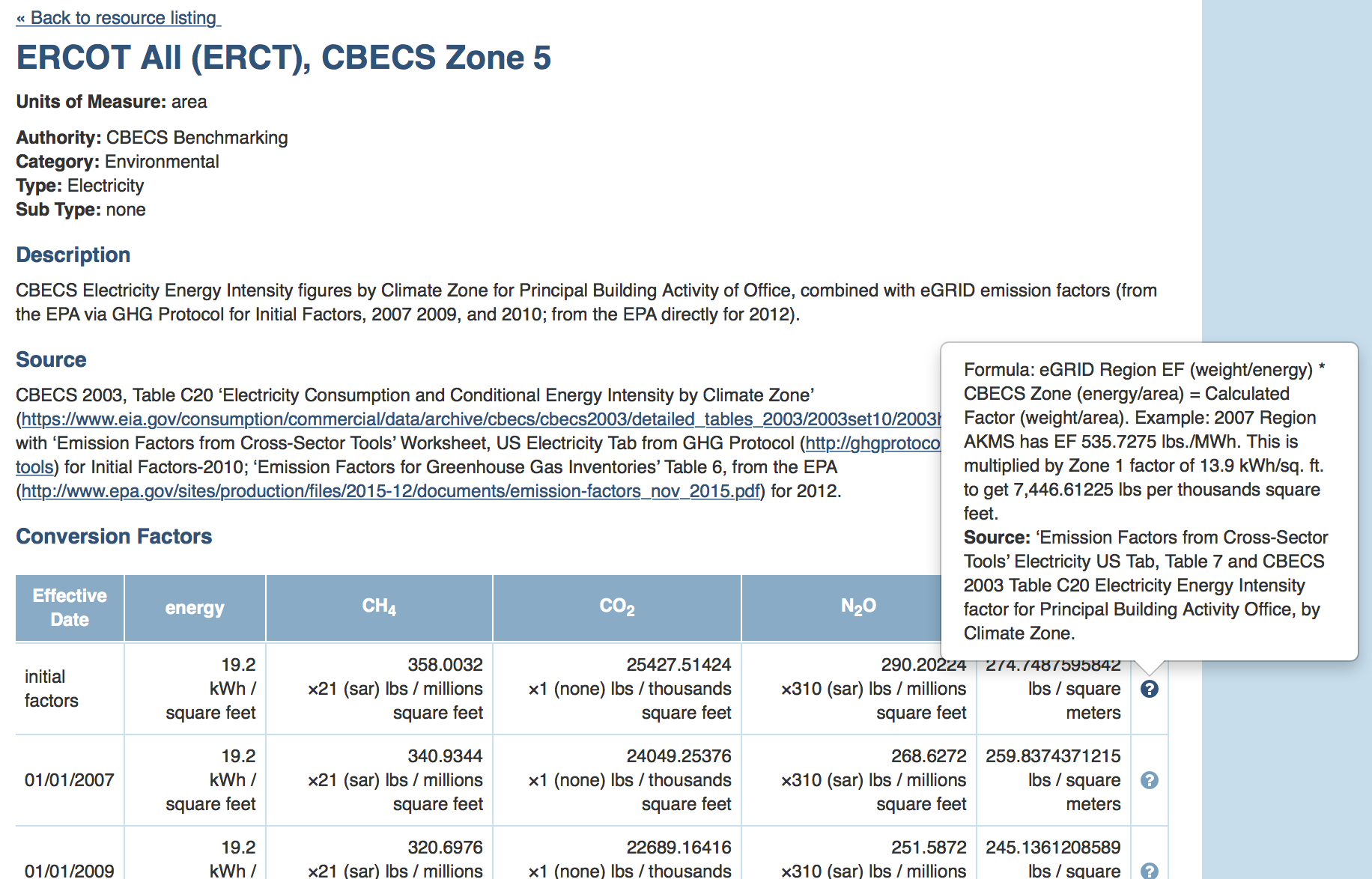In this case, a two-step conversion is applied. In the first step, an intermediate energy impact is calculated (using a benchmark EUI) from area activity units and tabulated in the first column. In the second step an emissions factor from the GHG Protocol is used to convert the energy caluclated in the first step, to emissions. This conversion methodology is described in the narrative.

#### Compact, Approximate Conversions for Individual Activity Records

In addition to the detailed conversion information on the resource page, users can quickly inspect approximate conversions on a record by record basis directly from a tracker's ledger. See the section titled Derivation of Impact Metrics in this article. An example is illustrated in the screenshot below: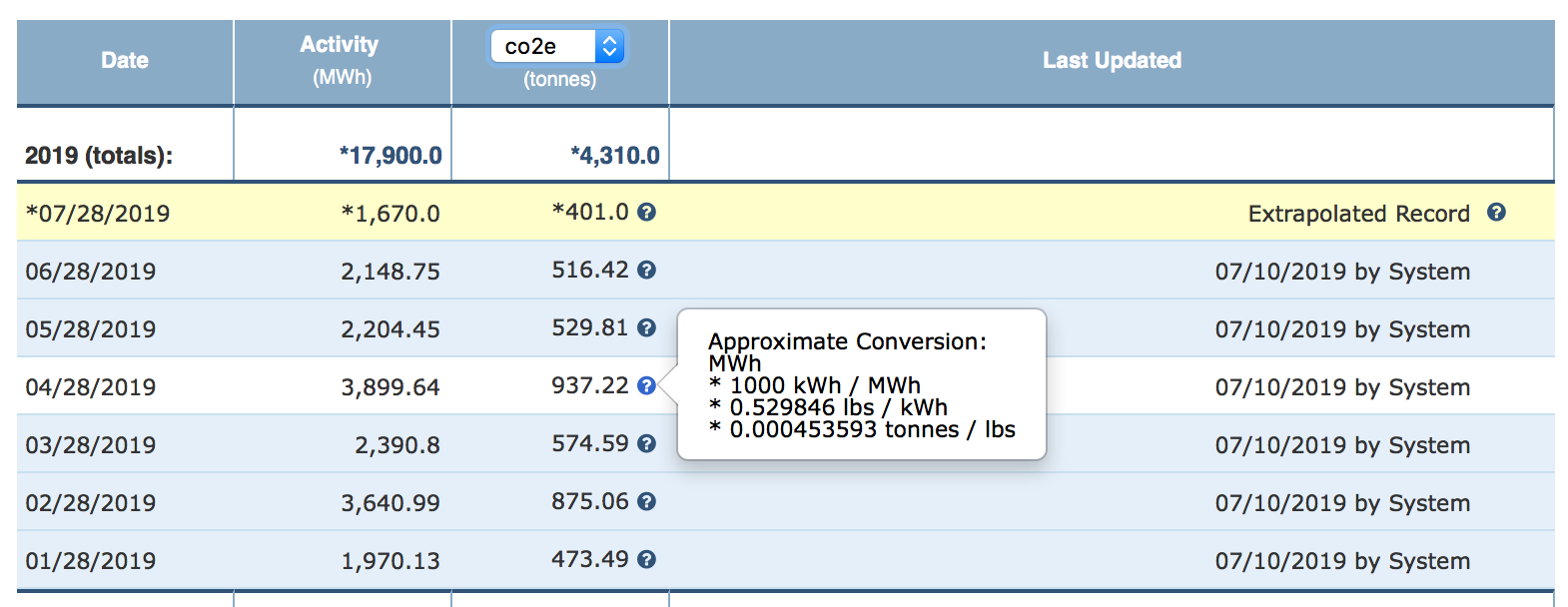Note that this view can be particularly useful because it also illustrates unit conversions.

#### Audit Trail

Although the audit trail functionality is focused more on temporal aggregation of activity data and proration the functionality can still be quite useful in understanding conversion of activity data to impact data. It is described in depth in this article and illustrated below.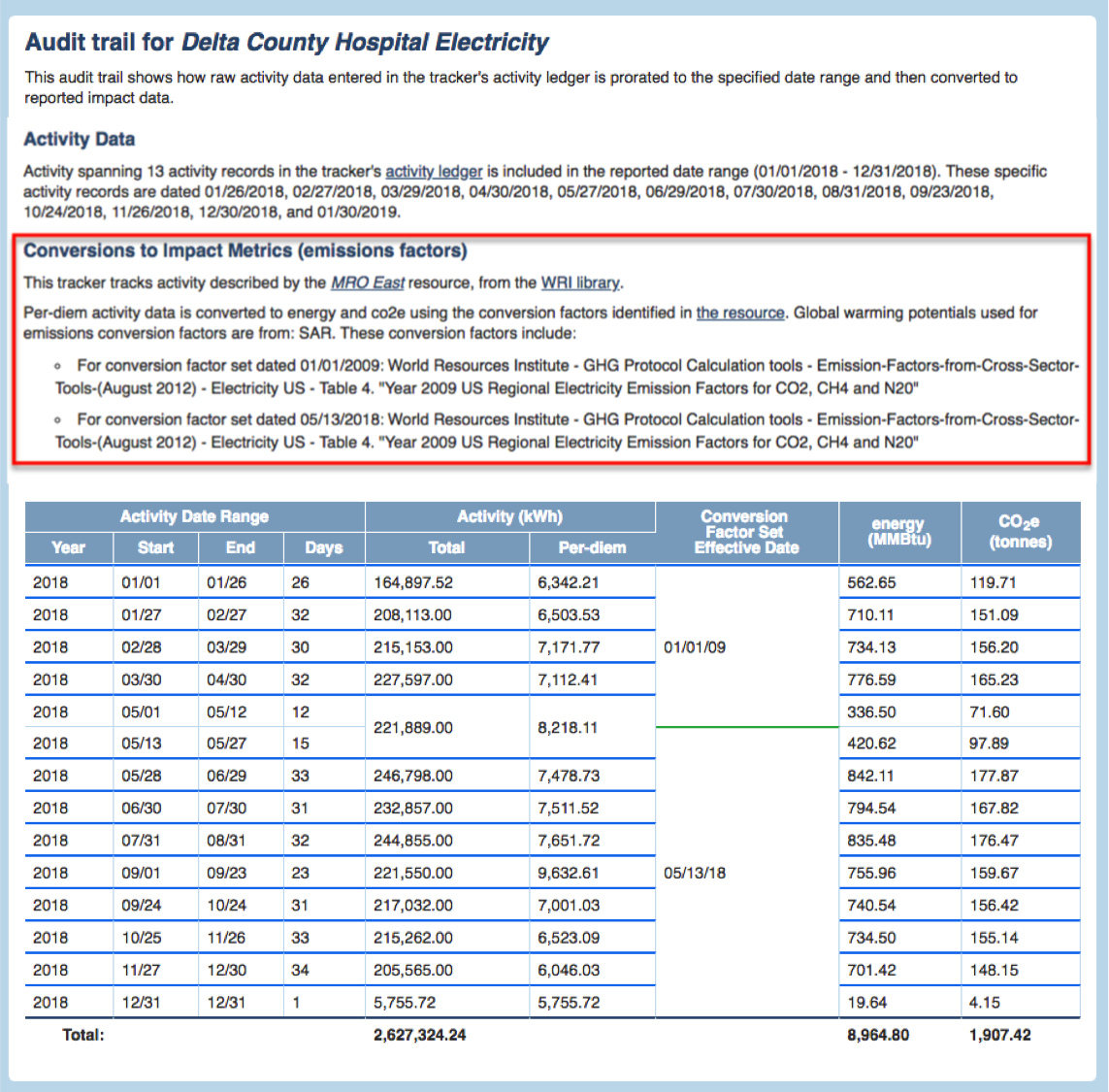### Example of Mobile Combustion Impact Calculation

Let's take a look at a calculation for emissions impacts resulting from mobile combustion activity. In this example, activity is expressed in distance units. The diagram below illustrates the conversion factor set for the Scope 5 Heavy Duty Vehicle - Articulated - Gasoline - Year 2005-present - US (by distance) resource. This resource corresponds to one of the entries in the GHG Protocol's list of Transport by Distance emissions factors.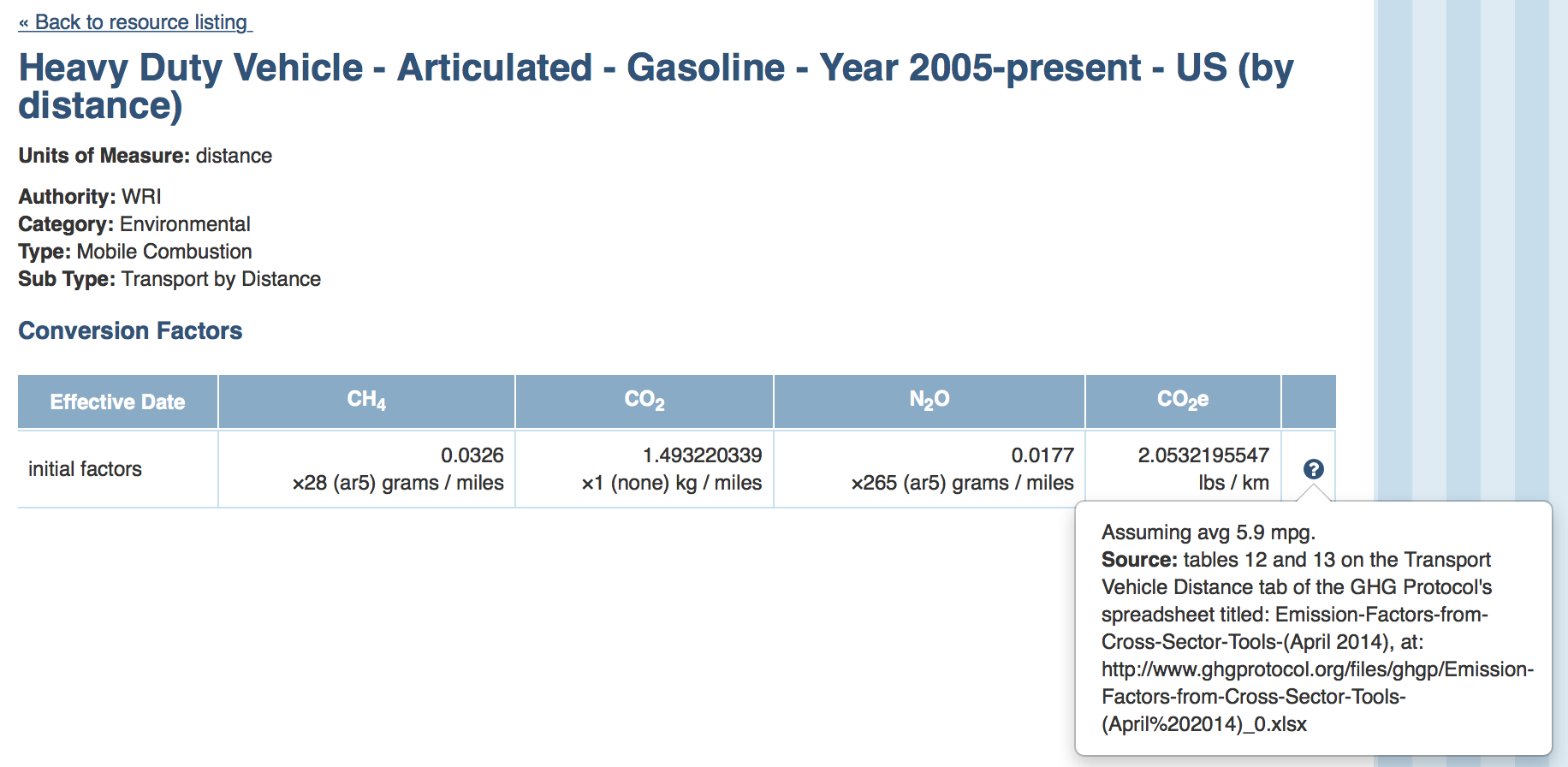This is another case of converting activity to CO2e using multiple emissions factors, similar to the one illustrated previously for electricity activity. The same formulas apply except that activity is expressed in distance units and therefore the emissions factors are expressed in weight of GHG per unit of distance. Note that Scope 5 performs any unit conversion required internally so while the emissions factors above may be expressed in miles, activity records may express distance in any standard unit of distance.

When reviewing conversions it's often helpful to refer to the Knowledge Base articles that describe the relevant resource library and to the tables identified in the Source (in this case, Tables 12 and 13 on the GHG Protocol's spreadsheet).

### Complex Mobile Combustion Calculation

The diagram below illustrates the data and considerations behind a complex mobile combustion conversion that incorporates several equations based on a mathematical model that quantifies mobile combustion emissions with a great deal of rigor and very fine granularity. In this conversion, several independent activity variables are combined to produce several impact metrics.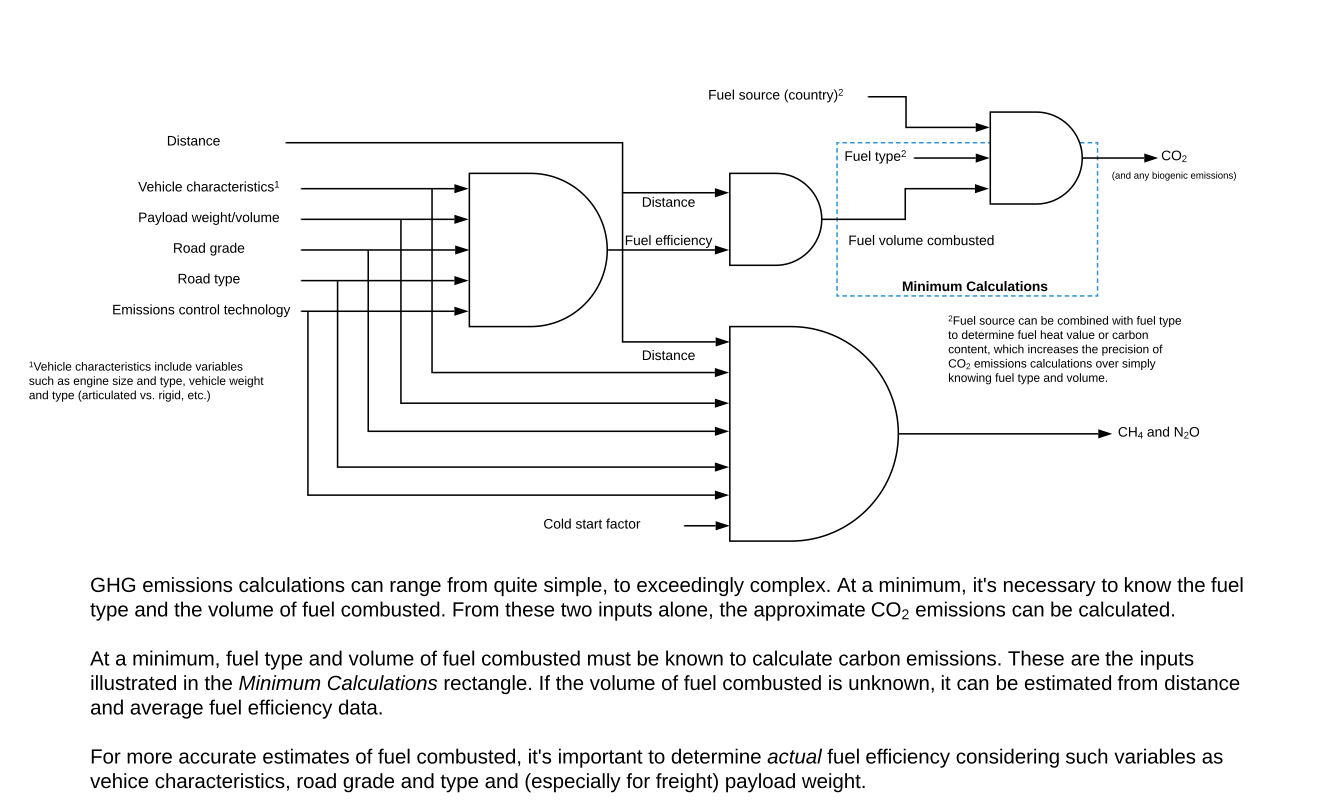We provide this example simply to show that some conversions can be quite complex. Such conversions are quite rare. They are typically developed for specific needs for specific users and are beyond the scope of this article. Most of the conversions required to report emissions consistent with current best practices are straightforward and follow either the multiple emissions factors or single emissions factor examples illustrated earlier in this article.

## Conclusion

Most Scope 5 conversions convert activity units to GHG metrics or energy. Most are quite simple; the activity is multiplied by a single emissions factor (in the form of weight of emissions per unit of activity) to produce a resulting weight of CO2e emissions.

In some variations,  component gases such as CH4 and N2O that contribute to the CO2e calculation are considered individually. In these cases, there is a separate emissions factor for each of the component gases. The component emissions are then multiplied by their GWPs and summed to produce total CO2e emissions.

In other variations, emissions factors may change over the period during which the activity was incurred. In these cases, the different emissions factors re applied on a prorated basis.

In some cases, multi step conversions are applied or formulas or lookup tables may be used.

All conversions are documented in various places in the application, the Knowledge Base or in documents referenced by the application.